# SAT Math Multiple Choice Question 194: Answer and Explanation

### Test Information

Question: 194

14.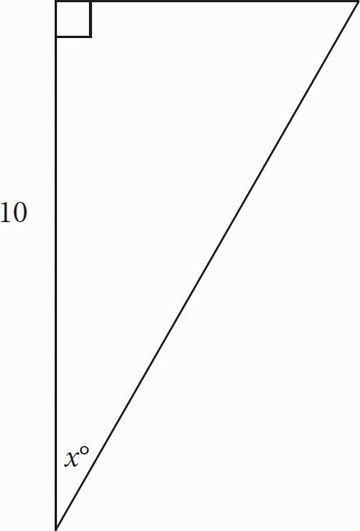In the figure above, sin x° =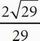. What is the perimeter of the figure?

• A. 10 +• B. 7 +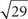• C. 14 + 2• D. 39 + 2C SOHCAHTOA tells you that sine is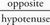, so if the side opposite the angle with measure x° is O and the hypotenuse is H, then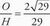. Solve for O, and you get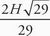. Now, you can use the Pythagorean theorem with the given side and substituting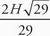for O in order to solve for H: 102 +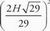= H2. Solve the exponents, and then reduce the fraction: 100 +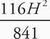= H2; 100 +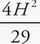= H2. Subtractfrom both sides: 100 =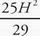. Divide both sides by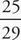, so 116 = H2. Square root both sides, and you find that H = 2.Using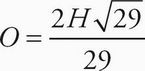from above, you can solve for O: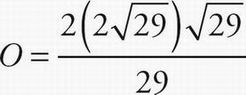= 4. Add the sides and you get 14 + 2, which is (C).